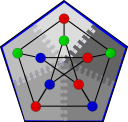Boost C++ Libraries

...one of the most highly regarded and expertly designed C++ library projects in the world.

This is the documentation for an old version of Boost. Click here to view this page for the latest version.PageRank

namespace graph {
template<typename Graph, typename RankMap, typename Done>
inline void
page_rank(const Graph& g, RankMap rank_map, Done done,
typename property_traits<RankMap>::value_type damping = 0.85);

template<typename Graph, typename RankMap>
inline void
page_rank(const Graph& g, RankMap rank_map);
}

The page_rank algorithm computes the ranking of vertices in a graph, based on the connectivity of a directed graph [PBMW98]. The idea of PageRank is based on a random model of a Web surfer, who starts a random web page and then either follows a link from that web page (choosing from the links randomly) or jumps to a completely different web page (not necessarily linked from the current page). The PageRank of each page is the probability of the random web surfer visiting that page.

Where Defined

<boost/graph/distributed/page_rank.hpp>

also accessible from

<boost/graph/page_rank.hpp>

Parameters

IN: Graph& g
The graph type must be a model of Distributed Vertex List Graph and Distributed Edge List Graph. The graph must be directed.
OUT: RankMap rank
Stores the rank of each vertex. The type RankMap must model the Read/Write Property Map concept and must be a distributed property map. Its key type must be the vertex descriptor of the graph type and its value type must be a floating-point or rational type.
IN: Done done

A function object that determines when the PageRank algorithm should complete. It will be passed two parameters, the rank map and a reference to the graph, and should return true when the algorithm should terminate.

Default: graph::n_iterations(20)

IN: typename property_traits<RankMap>::value_type damping

The damping factor is the probability that the Web surfer will select an outgoing link from the current page instead of jumping to a random page.

Default: 0.85

Complexity

Each iteration of PageRank requires O((V + E)/p) time on p processors and performs O(V) communication. The number of iterations is dependent on the input to the algorithm.

Bibliography

 [PBMW98] Lawrence Page, Sergey Brin, Rajeev Motwani, and Terry Winograd. The PageRank Citation Ranking: Bringing Order to the Web. Technical report, Stanford Digital Library Technologies Project, November 1998.

Copyright (C) 2005 The Trustees of Indiana University.

Authors: Douglas Gregor and Andrew Lumsdaine